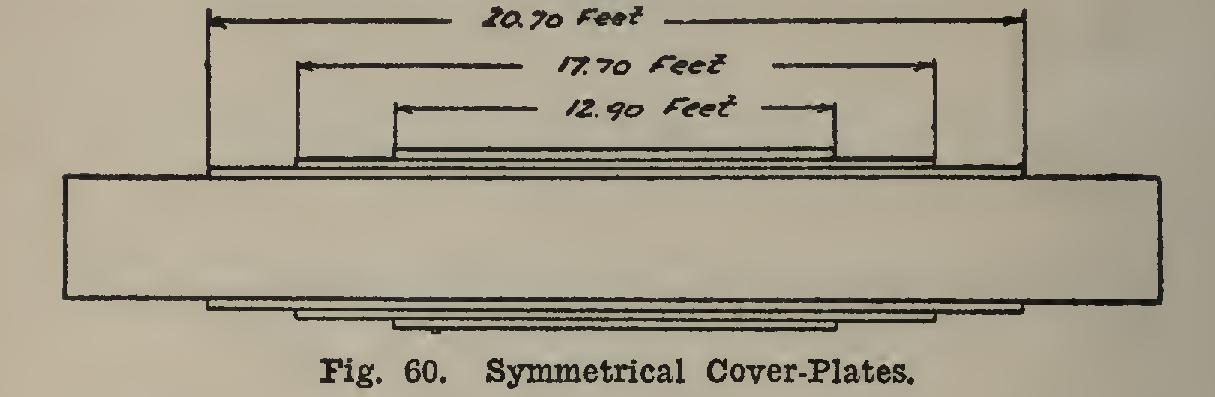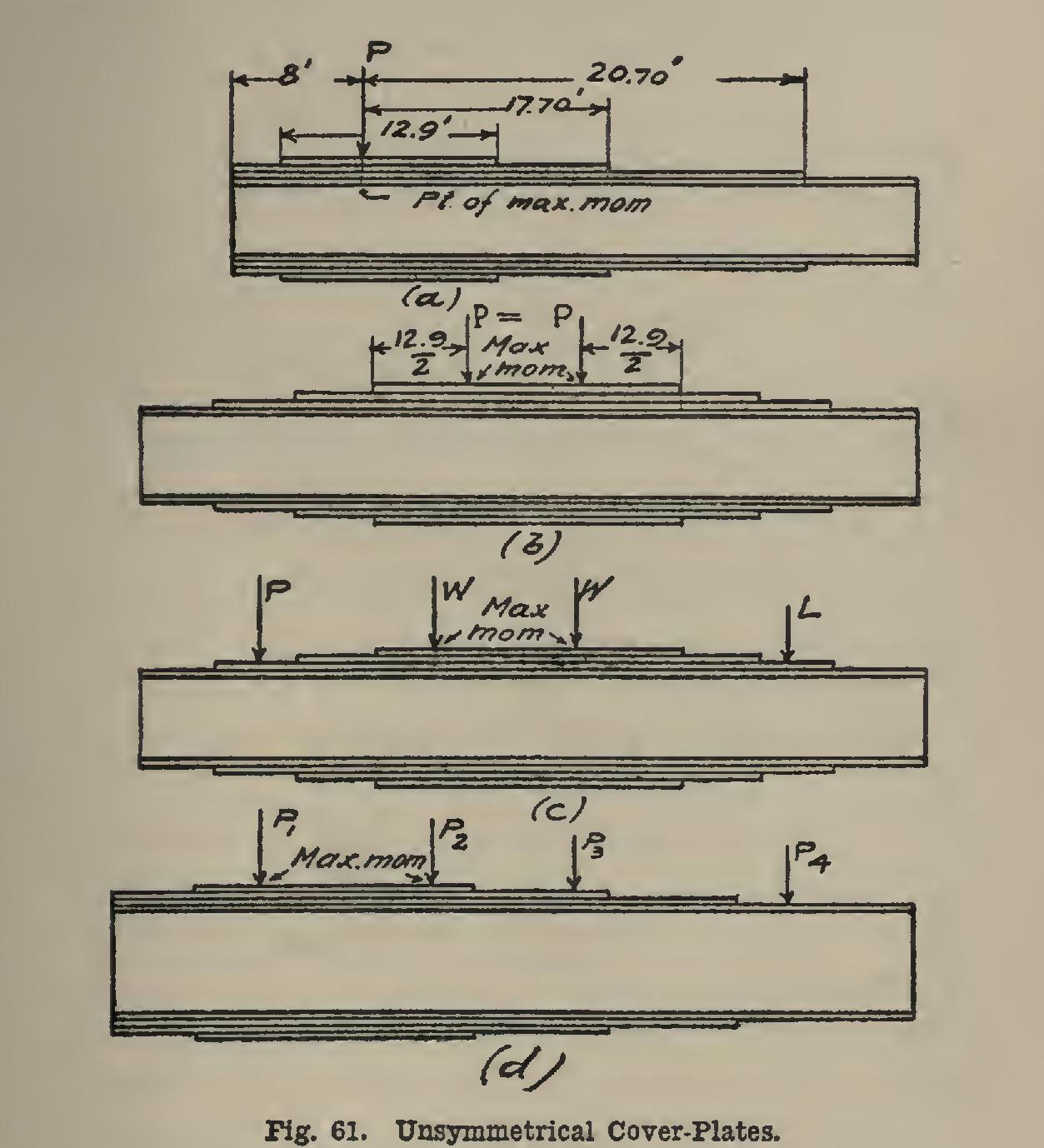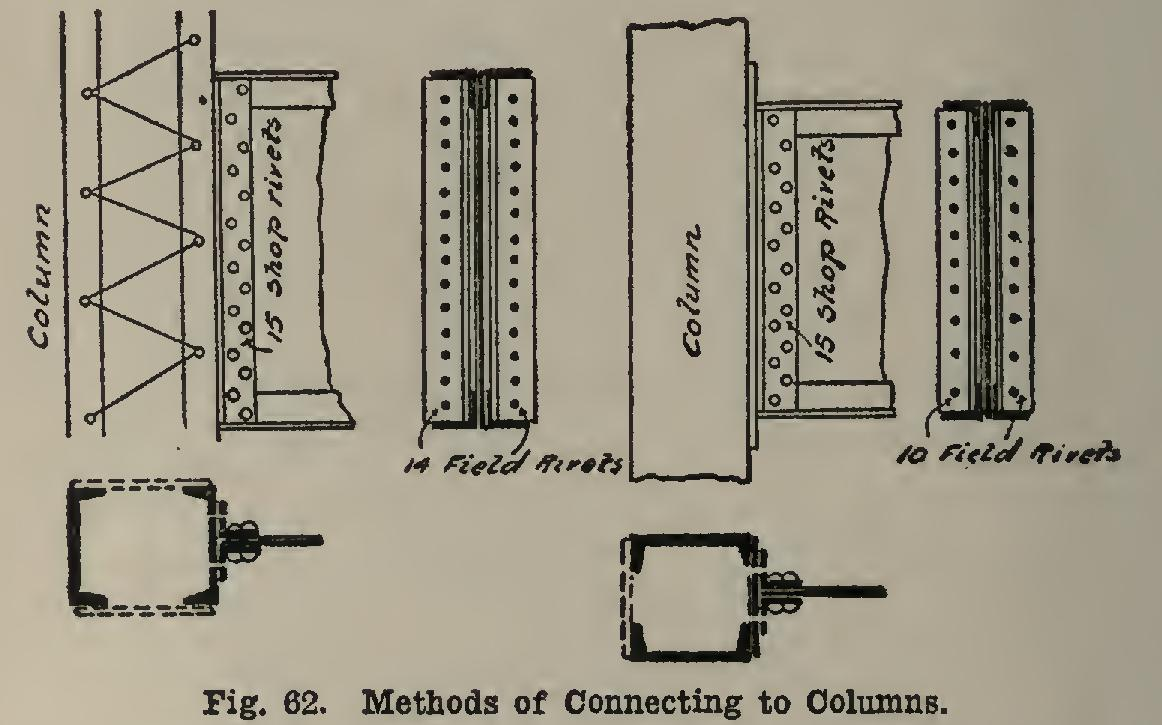# Girders 40

## plate, value, rivets, rivet, column, computed and web

Page: 1 2 3 4 5 6 7

The second cover-plate (see Fig. 59) has the same area as the first one. The total area from the bottom of plate 2 to the top of plate 1 is 3.75+3.75=7.50 (see p. 205 for rule); and the ratio for this plate length is: 7.50÷21.37=0.35. This requires, 6th column, a coefficient of 0.59; and the length of the second cover-plate is: Length=0.59x30=17.70 feet.

In a similar manner, the area of the cover plates above the bottom of the third cover-plate is computed to be 10.25 sq. in.; the ratio is com puted to be 0.48; the coefficient is computed to be 0.69; and the length of the cover-plate is 20.7 feet.

In case the moment was caused by a uniform load, or by a load at the middle, the cover-plates are arranged as shown in Fig. 60.

In case the moment is caused by concen trated loads placed certain distances each side of the center, the cover-plates must be placed as in Fig. 61, in order that the girder may be safe. The computing of the actual theoretical lengths for such cases is not within the scope of this work; but the method as shown will not be far from right, and it will be on the side of safety.From inspection of Figs. 60 and 61, the rule is seen to be that for any cover-plate the length as computed must extend half on each side of the point at which the maximum moment occurs. In case the point of maximum moment occurs closer to the end of the beam than one half the computed length of one of the cover plates, that cover-plate extends from the point in question to the end. If there are two points of maximum moment, or two points at which the moment is nearly equal, then the cover plates extend from each point toward the end an amount equal to half the length as computed; and they also extend between the loads (see b, Fig. 61).

42. Rivets in Girders. The girder may be joined to a column at the end, in which case a certain number of rivets are required. This number depends upon the unit-stress used, and upon the thickness of the material to which the column is connected.In (C 195) are given the bearing values of rivets for a wide range of allowable stresses. These values are for plates of different thick nesses, and the thicknesses are given in fractions of an inch. It is often desirable, as when a girder is joined to a channel or an I-beam, that the bear ing value of a rivet in the web of such a shape be known. Table XIII gives data from which this

value may be computed for and rivets.

As an example of the use of Table XIII, let it be required to determine the bearing value of a Winch rivet in the web of a 12-inch 25-pound channel, the allowable unit-stress for the rivet being 12,000 pounds. The thickness of the channel web is (C 101) 0.39 inch. For a 0.20-inch plate the value is 2,105 pounds; and since the difference between the 0.20-inch plate and the 0.39-inch channel web is 0.39-0.20= 0.19, the value of the rivet will be: Again, with same data as above, except that the channel is an 8-inch 13.75-pound, the thick ness of the web is 0.307 inch, and the difference between this and the 0.20-inch plate is 0.307 0.20=0.107 inch. Therefore the value of the rivet is: 1,-.2,105+10.7x105=3,229 pounds.

As explained on page 131, the rivet may fail by shear.

Rule—When the ends of a girder or beam are riveted to a plate or column of some form, (1) determine the bearing value of the rivet in the plate or web of column, and (2) compare it with the shearing value of a rivet (C 195). Divide the reaction at that end by the smallest of these values, and the result will be the num ber of rivets required if the riveting is done in the shop. If it is done in the field, add one-third more, and the result will be the required number.

Example—Reaction of girder is 87,500 pounds. The rivets are inch in diameter. The allowable unit-stresses are: Bearing 20,000; shear 10,000. The column is composed of two 10-inch 15-pound channels. The web of these channels is 0.24 inch thick. From Table XTIT, the bearing value of a rivet is Vb=3,504+175 (24-20)=4,204 pounds.

The shearing value (see column 4, table 3, C 195) is 6,010 pounds. Since the former is the smallest value, the number of shop rivets required would be: and the number of field rivets required would be: If, instead of the girder being connected to the column webs, it were connected to a plate inch thick, the rivets would be computed as follows: a *-inch plate (C 195, 3d table)=6,570 v in shear (C 195, 3d table)=6,010 Since the shear is the smallest, the rivets will tend to be cut off instead of tearing out of the plate, and the number required is 87,500±6,010 =15 shop; or field.Page: 1 2 3 4 5 6 7